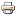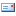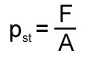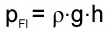# What is a hydraulic pressure dropPressure is a physical quantity that is measured in force per area. This means that the pressure indicates how much force (in N) is perpendicular to a surface.

### Static pressure

The static pressure is simply determined using the force per area formula as described above. This results in the following calculation formula:The illustration below illustrates the importance of pressure. In the picture you can see a liquid container which is closed (tightly) by a movable lid. A force F acts on the cover with the area A. This creates a pressure p in the container. In this simplified view, the pressure is the same at all points in the container.

### Position pressure / gravity pressure

The position pressure or gravity pressure depends on the point under consideration within a fluid (height), the density of the fluid and the acceleration due to gravity. It is the pressure that is created by the mass of the fluid and the acceleration of gravity. The position pressure / gravity pressure is calculated using the following formula:Here, ρ is the density in kg / m3, g is the acceleration due to gravity in m / s2, and h is the height in m.

The picture below makes it easier to understand the principle of position pressure. The picture shows a container in which the pressure is measured at two points at different levels. As the higher lying liquid presses on the underlying liquid, the pressure increases downwards. This means that the pressure at a higher point is lower than the pressure in the lower area of ​​the container.

### Dynamic pressure / back pressure

The dynamic pressure results from the kinetic energy of a flowing liquid on the surface of a body that is in this flow. The dynamic pressure is also referred to as dynamic pressure, since the flowing liquid accumulates on the corresponding body.
The dynamic pressure depends on the density of the liquid and its flow speed. It is calculated using the following formula:

pdyn = ρ / 2 . v2

Here, ρ is the density in kg / m3, and v is the speed (which goes into the calculation with the power of 2).

The illustration below shows the measurement of the dynamic pressure. A pipeline is shown in which a liquid flows. Another line with another liquid is in the flow. The liquid in the second tube is displaced by the flowing fluid. The height of the dynamic pressure can be measured and made visible via the height difference of the second liquid shown in green.

### Summary

In this script on the subject of hydraulics and fluid technology, the three types of hydraulic pressure were presented: Hydrostatic pressure, position pressure / gravity pressure and dynamic pressure or dynamic pressure.

As a rule, the dynamic pressure and the position pressure are negligible in hydraulic systems, since the static pressure is significantly higher.